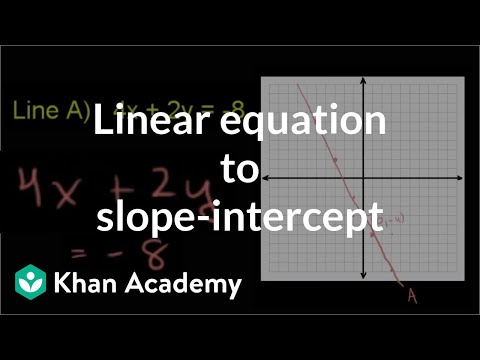Video

# Intro to intercepts (Full video)

Description: Learn what x and y-intercepts are. The equations used in this video are y = 0.5x - 3 and 5x + 6y = 30. So if we wanted to draw the line that represents the set of all points, all the coordinates where the x value and the y value satisfy this equation, we could start off by just trying to draw, by trying to draw a few of those points, and then connecting them with a line. Let's say when x is equal to zero, then you're gonna have 1/2 x 0 - 3, well then y is going to be -3. When x is equal to, let's try 4.

### Other videos you might be interested in### Ratio and Proportion in Grades 6-8: Connections to College and Career Skills (Full video)

#### The Hunt Institute